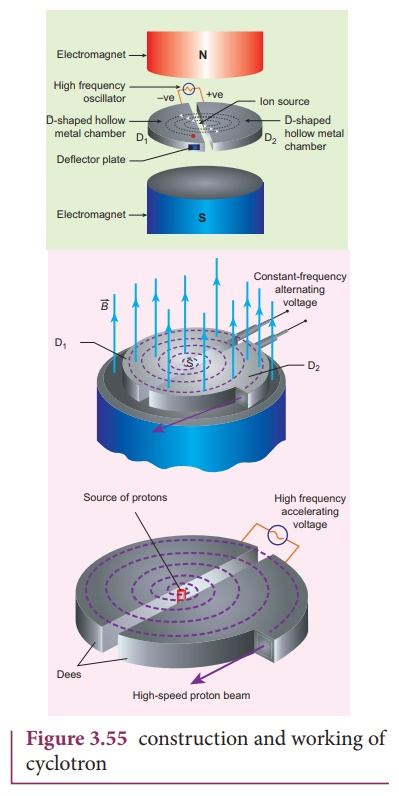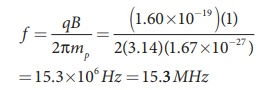Home | | Physics 12th Std | Cyclotron

# CyclotronCyclotron is a device used to accelerate the charged particles to gain large kinetic energy. It is also called as high energy accelerator. It was invented by Lawrence and Livingston in 1934.

Cyclotron

Cyclotron (Figure 3.54) is a device used to accelerate the charged particles to gain large kinetic energy. It is also called as high energy accelerator. It was invented by Lawrence and Livingston in 1934.

## Principle

When a charged particle moves normal to the magnetic field, it experiences magnetic Lorentz force.## Construction

The schematic diagram of a cyclotron is shown in Figure 3.55. The particles are allowed to move in between two semi-circular metal containers called Dees (hollow D - shaped objects). Dees are enclosed in an evacuated chamber and it is kept in a region with uniform magnetic field controlled by an electromagnet. The direction of magnetic field is normal to the plane of the Dees. The two Dees are kept separated with a gap and the source S (which ejects the particle to be accelerated) is placed at the center in the gap between the Dees. Dees are connected to high frequency alternating potential difference.## Working

Let us assume that the ion ejected from source S is positively charged. As soon as ion is ejected, it is accelerated towards a Dee (say, Dee – 1) which has negative potential at that time. Since the magnetic field is normal to the plane of the Dees, the ion undergoes circular path. After one semi-circular path in Dee-1, the ion reaches the gap between Dees. At this time, the polarities of the Dees are reversed so that the ion is now accelerated towards Dee-2 with a greater velocity. For this circular motion, the centripetal force of the charged particle q is provided by Lorentz force.From the equation (3.62), the increase in velocity increases the radius of circular path. This process continues and hence the particle undergoes spiral path of increasing radius. Once it reaches near the edge, it is taken out with the help of deflector plate and allowed to hit the target T.

Very important condition in cyclotron operation is the resonance condition. It happens when the frequency f at which the positive ion circulates in the magnetic field must be equal to the constant frequency of the electrical oscillator fosc

From equation (3.59), we haveThe time period of oscillation isThe kinetic energy of the charged particle is## Limitations of cyclotron

a)     the speed of the ion is limited

b)    electron cannot be accelerated

c)     uncharged particles cannot be accelerated

### EXAMPLE 3.26

Suppose a cyclotron is operated to accelerate protons with a magnetic field of strength 1 T. Calculate the frequency in which the electric field between two Dees could be reversed.

### Solution

Magnetic field B = 1 T

Mass of the proton, mp = 1.67 ×10−27 kg

Charge of the proton, q = 1.60 ×10−19 CTags : Principle, Construction, Working Principle, Limitations , 12th Physics : Magnetism and Magnetic Effects of Electric Current
Study Material, Lecturing Notes, Assignment, Reference, Wiki description explanation, brief detail
12th Physics : Magnetism and Magnetic Effects of Electric Current : Cyclotron | Principle, Construction, Working Principle, Limitations